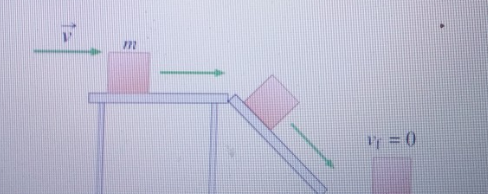# Problem: Find the amount of energy E dissipated by friction by the time the block stops.Express your answer in terms of some or all the variables m, v, and h and any appropriate constants.

###### FREE Expert Solution

From the law of conservation of energy:

$\overline{){\mathbf{P}}{\mathbf{.}}{{\mathbf{E}}}_{{\mathbf{i}}}{\mathbf{+}}{\mathbf{K}}{\mathbf{.}}{{\mathbf{E}}}_{{\mathbf{i}}}{\mathbf{=}}{\mathbf{P}}{\mathbf{.}}{{\mathbf{E}}}_{{\mathbf{f}}}{\mathbf{+}}{\mathbf{K}}{\mathbf{.}}{{\mathbf{E}}}_{{\mathbf{f}}}}$

The amount of energy E dissipated by friction by the time the block stops is the same as the initial total energy of the block with respect to the ground.

96% (1 ratings)###### Problem Details

Find the amount of energy E dissipated by friction by the time the block stops.

Express your answer in terms of some or all the variables m, v, and h and any appropriate constants.